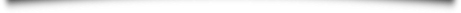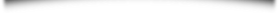GUTENBERG COLLEGE ONLINE CATALOG

# Mathematics

We human beings are rational creatures and as such desire to put our experience into some sort of rational order. Mathematics is one way in which we have pursued that goal. Mathematics is a formal exploration of the nature and limitations of our rationality as it relates to quantity. To the extent that our understanding of rationality has changed over time, so has our understanding of mathematics.

The mathematics curriculum has a dual purpose in examining the nature of mathematical reasoning. First, an examination of the nature and limitations of our rationality is important in its own right. An understanding of rationality logically precedes a pursuit of knowledge and is thus a worthwhile goal. Second, the development of mathematics tells us something of our culture’s ideas and their origins.

The two-year sequence is divided into ancient and modern mathematics. The first year is devoted to ancient mathematics, primarily examining Euclid’s Elements. The second year covers modern mathematics, tracing the development of algebra and calculus and examining questions raised at the turn of the twentieth century about the foundations of mathematics.

## Ancient Mathematics (MTH 101-103)

In their freshman year, students master proofs from the thirteen books of Euclid’s Elements. The subject matter begins with standard plane geometry in books one, three, and four, together with a bit of algebraic geometry (the exploration of algebraic types of problems using geometrical constructions) in book two. Students then consider the theory of proportion in book five and its application to plane geometry (book six) and number theory (books seven and ten). The year concludes with a study of the method of exhaustion (the forerunner of calculus) and its application to the measurement of the circle, first by Euclid in the Elements and then by Archimedes.

## Modern Mathematics (MTH 201-203)

In the first quarter of the sophomore year, students examine the development of algebra. They read portions of works by Diophantus, Viéte, and Descartes. In their second quarter, students focus on the study of the infinite and infinitesimal—in other words, calculus. They study Zeno’s paradoxes, Newton, Berkeley, Cauchy, and Dedekind. In their final quarter, students consider the modern developments of Cantor, Frege, and Gödel—the emphasis here being the foundations of mathematical thought.Gutenberg Catalog Contents

## Financial Aid